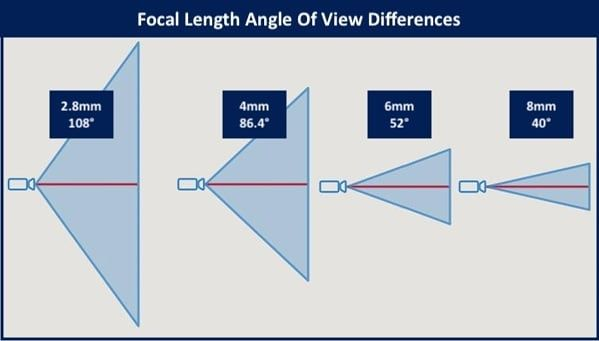When working with cameras, the distance from the camera has a significant impact on image quality. As you get further from a camera, the field of view (i.e. the width from the left to right edges of the camera image) gets larger and larger. This occurs because the camera lens collects light over what is known as the Angle of View (AOV). The following image describes this best.This image allows you to visualize two things:

• At the same distance from the camera, the width of the scene gets larger as the AOV increases.
• Because camera resolution is constant, this has the effect of reducing pixel density (the number of pixels per foot) as the angle increases.
• At the same camera angle, as you get further from the camera, the width of the scene gets larger.
• Because camera resolution is constant, this has the effect of reducing pixel density as the distance increases.

Pixel density is very important for face recognition. The higher the pixel density, the more detail that's captured in a face image and thus the better face recognition performs.

Camera Specs Affecting Angle of View

The AOV can be computed by knowing the focal length and image sensor size as explained here or here.

• Sensor Size - The width of the image sensor on the camera, typically given in inches as a fraction such as 1/2.8".
• Focal Length - The distance (in mm) from the lens to the camera's image sensor.

It's important to note that a lens and image sensor are separate components in the camera. You can change lenses on some cameras. The lens alone doesn't determine the AOV; you must know the specs for the lens and the camera to obtain the AOV.

Computing the Angle of View

Only try to compute the AOV if you have searched and been unable to find the AOV in the camera specifications.

The Easy Way

There are good online tools to compute the AOV from the image sensor size. For example, IPVM offers a camera calculator. It has a library of standard cameras so it's very useful for camera selection. If you select the Generic Camera (default when adding new camera) then you can compute the hAOV or focal length given a fixed sensor size.  The tool is shown below. It even shows how the changes impact the scene images captured by the camera.The Hard Way

You can compute the angle of view by using the following formula:Where:

• AFOV is the angular field of view in degrees. (Note: Excel and some calculators will give results for the tangent (tan) function in radians. This should be converted to degrees if your result is an integer between 0 to 2.
• h is the image sensor width in millimeters. (Convert from inches to mm using <inches> x 25.4)
• f is the focal length in mm. (Most lenses report this value and it's often written on the side of the lens.)